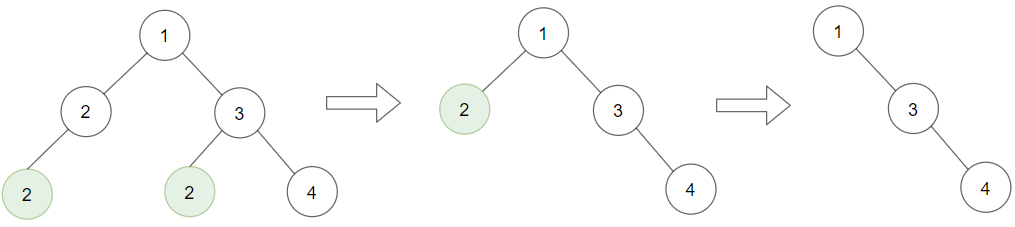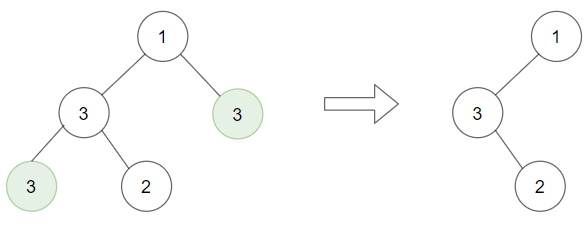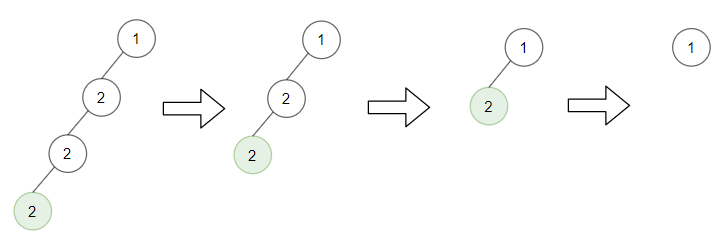# GeetCode Hub

Given a binary tree `root` and an integer `target`, delete all the leaf nodes with value `target`.

Note that once you delete a leaf node with value `target`if it's parent node becomes a leaf node and has the value `target`, it should also be deleted (you need to continue doing that until you can't).

Example 1:```Input: root = [1,2,3,2,null,2,4], target = 2
Output: [1,null,3,null,4]
Explanation: Leaf nodes in green with value (target = 2) are removed (Picture in left).
After removing, new nodes become leaf nodes with value (target = 2) (Picture in center).
```

Example 2:```Input: root = [1,3,3,3,2], target = 3
Output: [1,3,null,null,2]
```

Example 3:```Input: root = [1,2,null,2,null,2], target = 2
Output: 
Explanation: Leaf nodes in green with value (target = 2) are removed at each step.
```

Example 4:

```Input: root = [1,1,1], target = 1
Output: []
```

Example 5:

```Input: root = [1,2,3], target = 1
Output: [1,2,3]
```

Constraints:

• `1 <= target <= 1000`
• The given binary tree will have between `1` and `3000` nodes.
• Each node's value is between `[1, 1000]`.

/** * Definition for a binary tree node. * public class TreeNode { * int val; * TreeNode left; * TreeNode right; * TreeNode() {} * TreeNode(int val) { this.val = val; } * TreeNode(int val, TreeNode left, TreeNode right) { * this.val = val; * this.left = left; * this.right = right; * } * } */ class Solution { public TreeNode removeLeafNodes(TreeNode root, int target) { } }